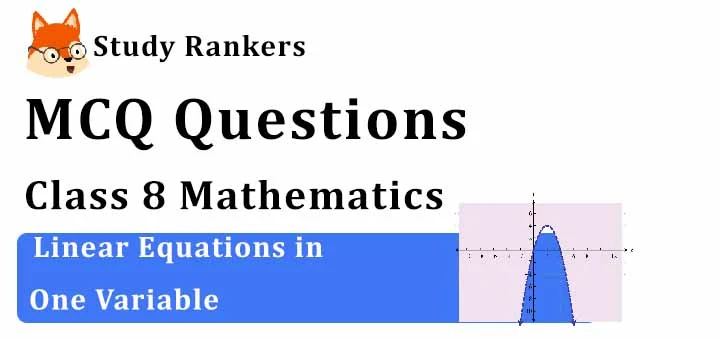## MCQ Questions for Class 8 Maths: Ch 2 Linear Equations in One Variable1. David cuts a bread into two equal pieces and cuts one half into smaller pieces of equal size. Each of the small pieces is twenty grams in weight. If he has seven pieces of the bread all with him, how heavy is the original cake.
(a) 120 gm
(b) 180 gm
(c) 300 gm
(d) 240 gm
► (d) 240 gm

2. Mary was counting down from 34 and Thomas was counting upwards simultaneously, the number starting from 1 and he was calling out only the odd numbers. Which common number will they call out at the same time if they were calling out at the same speed?
(a) 20
(b) 21
(c) 22
(d) 23
► (d) 23

3. Find the solution of 2x - 3 = 7
(a) 3
(b) 4
(c) 5
(d) none of these
► (c) 5

4. The numerator of a fraction is 4 less than the denominator. If the numerator is decreased by 2 and denominator is increased by 1, then the denominator is eight times the numerator. Find the fraction.
(a) 4/12
(b) 3/13
(c) 3/7
(d) 11/7
► (c) 3/7

5. Solve 2x − 3 = x + 2
(a) 4
(b) 5
(c) 3
(d) 0
► (b) 5

6. In the following number sequence, how many such even numbers are there which are exactly divisible by its immediate preceding number but not exactly divisible by its immediate following number?
3  8  4  1  5  7  2  8  3  4  8  9  3  9  4  2  1  5  8  2
(a) One
(b) Two
(c) Three
(d) Four
► (b) Two

7. Amina thinks of a number and subtracts 5/2 from it. She multiplies the result by 8. The result now obtained is 3 times the same number she thought of. What is the number?
(a) 2
(b) 3
(c) 4
(d) none of these
► (c) 4

8. The sum of three consecutive multiples of 7 is 357. Find the smallest multiple.
(a) 112
(b) 126
(c) 119
(d) 116
► (a) 112

9. Solve: 5x−2(2x−7)=(3x−1)+7/2
(a) 2
(b) 3
(c) 1/2
(d) 23/4
► (d) 23/4

10. The distance between two mile stones is 230 km and two cars start simultaneously from the milestones in opposite directions and the distance between them after three hours is 20 km. If the speed of one car is less than that of other by 10 km/h, find the speed of each car.
(a) 25 km/h, 40 km/h
(b) 40 km/h, 50 km/h
(c) 20 km/h, 40 km/h
(d) 30 km/h, 40 km/h
► (d) 30 km/h, 40 km/h

11. The difference between two whole numbers is 66. The ratio of the two numbers is 2 : 5. What are the two numbers?
(a) 22 and 88
(b) 44 and 66
(c) 44 and 110
(d) 33 and 99
► (c) 44 and 110

12. In an equation the values of the expressions on the LHS and RHS are _______.
(a) different
(b) not equal
(c) equal
(d) none of these
► (c) equal

13. The digits of a two-digit number differ by 3. If the digits are interchanged, and the resulting number is added to the original number, we get 143. What can be the original number?
(a) 85
(b) 58
(c) 36
(d) 76
► (a) 85

14. Solve 2y + 9 = 4.
(a) -5/2
(b) 1/2
(c) 2
(d) none of these
► (a) -5/2

15. Solve: 7x = 21
(a) 3
(b) 2
(c) 14
(d) none of these
► (a) 3

16. An MNC company employed 25 men to do the official work in 32 days. After 16 days, it employed 5 more men and work was finished one day earlier. If it had not employed additional men, it would have been behind by how many days?
(a) 1 day
(b) 2 days
(c) 3 days
(d) 2.5 days
► (b) 2 days

17. The sum of two digit number and the number formed by interchanging its digit is 110. If ten is subtracted from the first number, the new number is 4 more than 5 times of the sum of the digits in the first number. Find the first number.
(a) 46
(b) 48
(c) 64
(d) 84
► (c) 64

18. Aruna cut a cake into two halves and cuts one half into smaller pieces of equal size. Each of the small pieces is twenty grams in weight. If she has seven pieces of the cake in all with her, how heavy was the original cake ?
(a) 120 gm
(b) 180 gm
(c) 300 gm
(d) 240 gm
► (d) 240 gm

19. Sum of two numbers is 95. If one exceeds the other by 15, find the numbers.
(a) 40 and 60
(b) 50 and 55
(c) 50 and 60
(d) 40 and 55
► (d) 40 and 55

20. Solve: y + 3 = 10
(a) 13
(b) 7
(c) -7
(d) none of these
► (b) 7

21. Solve: 3x = 12
(a) 15
(b) 4
(c) 9
(d) 3
► (b) 4

22. What will be the solution of these equations ax+by = a-b, bx-ay = a+b
(a) x = 1, y = 2
(b) x = 2,y = -1
(c) x = -2, y = -2
(d) x = 1, y = -1
► (d) x = 1, y = -1